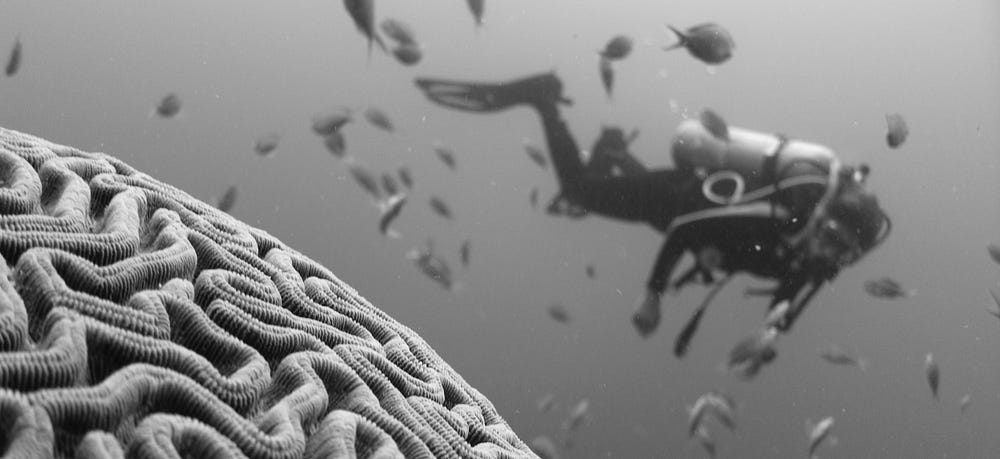# [Memo Sheet] Deep Neural Network

Source: Deep Learning on MediumHave you ever dreamed of a place where the key points for understanding neural networks would be condensed?
A place where you can easily find out what’s under the hood of learning/deep learning machine models? This [Memo Sheet] series of articles could make your dreams come true 💫 Main equations, simple model implementation, domain vocabulary and little tips are summarised for you!

# Content

This article focuses on describing, step by step, the architecture of a classical neural network known as multilayer perceptron. This article is not intended to be exhaustive but is a kind of reminder for those who would like to refresh their memory or clarify their ideas.

1. Brief History of the Birth of Neural Networks
2. Logistic/Neural Unit
3. Neural Network
4. Deep Neural Network

# Brief History of the Birth of Neural Network

The origin of artificial neural networks dates back to the 1950s. At that time, utopians in artificial intelligence embraced learning theories developed in the field of neuroscience. In particular, the role of neural inter-connections in learning became a source of inspiration for computer researchers. In 1957, the psychologist Frank Rosenblatt built the perceptron, the first learning machine which remains a reference model in machine learning. The perceptron, composed of a single layer of artificial neurons, showed limited capabilities. Researchers tried to improve it by introducing several layers of neurons but did not find an adequate learning algorithm. Research on neural networks then declined until the 1980s. At this time, the method of gradient back-propagation became popular and allowed the training of multilayer neural networks (multilayer perceptron). Although this field of research was not referred to by this term at the time, deep learning was born! Since then, other types of neural networks have emerged: convolutional neural networks, recurrent neural networks, generative adversarial networks, to name a few.

# Logistic/Neural Unit

The logistic or neural unit can be seen as a very simplified model of a neuron. It is the basic brick to build a neural network. It takes a set of n inputs/features and computes a scalar number, known as activation, based on the weight (W) and bias (b) parameters. The computation of the output is named forward propagation and is computed as follows:

1. Linear: weighted sum of the inputs x. The results is stored into a variable z.

2. Activation: apply a non linear function to z.

The activation can be sigmoid, tanh, ReLU or even the identity function for the output of a regression network. To refresh your memory on the activation functions, you can have a look at this article.
Below is a memo sheet of a logistic unit with its associated equations. A single training example x is considered here. The computation final output, ŷ, is the predicted class or output value.